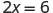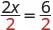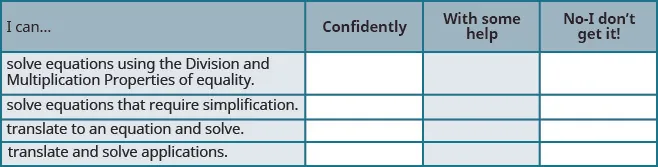Elementary Algebra

# 2.2Solve Equations using the Division and Multiplication Properties of Equality

Elementary Algebra2.2 Solve Equations using the Division and Multiplication Properties of Equality

### Learning Objectives

By the end of this section, you will be able to:

• Solve equations using the Division and Multiplication Properties of Equality
• Solve equations that require simplification
• Translate to an equation and solve
• Translate and solve applications

### Be Prepared 2.2

Before you get started, take this readiness quiz.

1. Simplify: $−7(1−7).−7(1−7).$
If you missed this problem, review Example 1.68.
2. Evaluate $9x+29x+2$ when $x=−3x=−3$.
If you missed this problem, review Example 1.57.

### Solve Equations Using the Division and Multiplication Properties of Equality

You may have noticed that all of the equations we have solved so far have been of the form $x+a=bx+a=b$ or $x−a=bx−a=b$. We were able to isolate the variable by adding or subtracting the constant term on the side of the equation with the variable. Now we will see how to solve equations that have a variable multiplied by a constant and so will require division to isolate the variable.

Let’s look at our puzzle again with the envelopes and counters in Figure 2.5.

Figure 2.5 The illustration shows a model of an equation with one variable multiplied by a constant. On the left side of the workspace are two instances of the unknown (envelope), while on the right side of the workspace are six counters.

In the illustration there are two identical envelopes that contain the same number of counters. Remember, the left side of the workspace must equal the right side, but the counters on the left side are “hidden” in the envelopes. So how many counters are in each envelope?

How do we determine the number? We have to separate the counters on the right side into two groups of the same size to correspond with the two envelopes on the left side. The 6 counters divided into 2 equal groups gives 3 counters in each group (since $6÷2=36÷2=3$).

What equation models the situation shown in Figure 2.6? There are two envelopes, and each contains $xx$ counters. Together, the two envelopes must contain a total of 6 counters.

Figure 2.6 The illustration shows a model of the equation $2x=62x=6$.If we divide both sides of the equation by 2, as we did with the envelopes and counters,we get:We found that each envelope contains 3 counters. Does this check? We know $2·3=62·3=6$, so it works! Three counters in each of two envelopes does equal six!

This example leads to the Division Property of Equality.

### The Division Property of Equality

For any numbers a, b, and c, and $c≠0c≠0$,

$Ifa=b,thenac=bcIfa=b,thenac=bc$

When you divide both sides of an equation by any non-zero number, you still have equality.

### Manipulative Mathematics

Doing the Manipulative Mathematics activity “Division Property of Equality” will help you develop a better understanding of how to solve equations by using the Division Property of Equality.

The goal in solving an equation is to ‘undo’ the operation on the variable. In the next example, the variable is multiplied by 5, so we will divide both sides by 5 to ‘undo’ the multiplication.

### Example 2.13

Solve: $5x=−27.5x=−27.$

### Try It 2.25

Solve: $3y=−41.3y=−41.$

### Try It 2.26

Solve: $4z=−55.4z=−55.$

Consider the equation $x4=3x4=3$. We want to know what number divided by 4 gives 3. So to “undo” the division, we will need to multiply by 4. The Multiplication Property of Equality will allow us to do this. This property says that if we start with two equal quantities and multiply both by the same number, the results are equal.

### The Multiplication Property of Equality

For any numbers a, b, and c,

$Ifa=b,thenac=bcIfa=b,thenac=bc$

If you multiply both sides of an equation by the same number, you still have equality.

### Example 2.14

Solve: $y−7=−14.y−7=−14.$

### Try It 2.27

Solve: $a−7=−42.a−7=−42.$

### Try It 2.28

Solve: $b−6=−24.b−6=−24.$

### Example 2.15

Solve: $−n=9.−n=9.$

### Try It 2.29

Solve: $−k=8.−k=8.$

### Try It 2.30

Solve: $−g=3.−g=3.$

### Example 2.16

Solve: $34x=12.34x=12.$

### Try It 2.31

Solve: $25n=14.25n=14.$

### Try It 2.32

Solve: $56y=15.56y=15.$

In the next example, all the variable terms are on the right side of the equation. As always, our goal in solving the equation is to isolate the variable.

### Example 2.17

Solve: $815=−45x.815=−45x.$

### Try It 2.33

Solve: $925=−45z.925=−45z.$

### Try It 2.34

Solve: $56=−83r.56=−83r.$

### Solve Equations That Require Simplification

Many equations start out more complicated than the ones we have been working with.

With these more complicated equations the first step is to simplify both sides of the equation as much as possible. This usually involves combining like terms or using the distributive property.

### Example 2.18

Solve: $14−23=12y−4y−5y.14−23=12y−4y−5y.$

### Try It 2.35

Solve: $18−27=15c−9c−3c.18−27=15c−9c−3c.$

### Try It 2.36

Solve:$18−22=12x−x−4x.18−22=12x−x−4x.$

### Example 2.19

Solve: $−4(a−3)−7=25.−4(a−3)−7=25.$

### Try It 2.37

Solve: $−4(q−2)−8=24.−4(q−2)−8=24.$

### Try It 2.38

Solve: $−6(r−2)−12=30.−6(r−2)−12=30.$

Now we have covered all four properties of equality—subtraction, addition, division, and multiplication. We’ll list them all together here for easy reference.

### Properties of Equality

$Subtraction Property of EqualityAddition Property of Equality For any real numbersa,b,andc,For any real numbersa,b,andc, ifa=b,thena−c=b−c.ifa=b,thena+c=b+c. Division Property of EqualityMultiplication Property of Equality For any numbersa,b,andc,andc≠0,For any numbersa,b,andc, ifa=b,thenac=bc.ifa=b, thenac=bc.Subtraction Property of EqualityAddition Property of Equality For any real numbersa,b,andc,For any real numbersa,b,andc, ifa=b,thena−c=b−c.ifa=b,thena+c=b+c. Division Property of EqualityMultiplication Property of Equality For any numbersa,b,andc,andc≠0,For any numbersa,b,andc, ifa=b,thenac=bc.ifa=b, thenac=bc.$

When you add, subtract, multiply, or divide the same quantity from both sides of an equation, you still have equality.

### Translate to an Equation and Solve

In the next few examples, we will translate sentences into equations and then solve the equations. You might want to review the translation table in the previous chapter.

### Example 2.20

Translate and solve: The number 143 is the product of $−11−11$ and y.

### Try It 2.39

Translate and solve: The number 132 is the product of −12 and y.

### Try It 2.40

Translate and solve: The number 117 is the product of −13 and z.

### Example 2.21

Translate and solve: $nn$ divided by 8 is $−32−32$.

### Try It 2.41

Translate and solve: $nn$ divided by 7 is equal to $−21−21$.

### Try It 2.42

Translate and solve: $nn$ divided by 8 is equal to $−56−56$.

### Example 2.22

Translate and solve: The quotient of $yy$ and $−4−4$ is $6868$.

### Try It 2.43

Translate and solve: The quotient of $qq$ and $−8−8$ is 72.

### Try It 2.44

Translate and solve: The quotient of $pp$ and $−9−9$ is 81.

### Example 2.23

Translate and solve: Three-fourths of $pp$ is 18.

### Try It 2.45

Translate and solve: Two-fifths of $ff$ is 16.

### Try It 2.46

Translate and solve: Three-fourths of $ff$ is 21.

### Example 2.24

Translate and solve: The sum of three-eighths and $xx$ is one-half.

### Try It 2.47

Translate and solve: The sum of five-eighths and x is one-fourth.

### Try It 2.48

Translate and solve: The sum of three-fourths and x is five-sixths.

### Translate and Solve Applications

To solve applications using the Division and Multiplication Properties of Equality, we will follow the same steps we used in the last section. We will restate the problem in just one sentence, assign a variable, and then translate the sentence into an equation to solve.

### Try It 2.50

Translate and solve:

### Try It 2.51

Translate and solve:

The annual property tax on the Mehta’s house is $1,800, calculated as $151,000151,000$ of the assessed value of the house. What is the assessed value of the Mehta’s house? ### Try It 2.52 Translate and solve: Stella planted 14 flats of flowers in $2323$ of her garden. How many flats of flowers would she need to fill the whole garden? ### Section 2.2 Exercises #### Practice Makes Perfect Solve Equations Using the Division and Multiplication Properties of Equality In the following exercises, solve each equation using the Division and Multiplication Properties of Equality and check the solution. 77. $8 x = 56 8 x = 56$ 78. $7 p = 63 7 p = 63$ 79. $−5 c = 55 −5 c = 55$ 80. $−9 x = −27 −9 x = −27$ 81. $−809 = 15 y −809 = 15 y$ 82. $−731 = 19 y −731 = 19 y$ 83. $−37 p = −541 −37 p = −541$ 84. $−19 m = −586 −19 m = −586$ 85. $0.25 z = 3.25 0.25 z = 3.25$ 86. $0.75 a = 11.25 0.75 a = 11.25$ 87. $−13 x = 0 −13 x = 0$ 88. $24 x = 0 24 x = 0$ 89. $x 4 = 35 x 4 = 35$ 90. $z 2 = 54 z 2 = 54$ 91. $−20 = q −5 −20 = q −5$ 92. $c −3 = −12 c −3 = −12$ 93. $y 9 = −16 y 9 = −16$ 94. $q 6 = −38 q 6 = −38$ 95. $m −12 = 45 m −12 = 45$ 96. $−24 = p −20 −24 = p −20$ 97. $− y = 6 − y = 6$ 98. $− u = 15 − u = 15$ 99. $− v = −72 − v = −72$ 100. $− x = −39 − x = −39$ 101. $2 3 y = 48 2 3 y = 48$ 102. $3 5 r = 75 3 5 r = 75$ 103. $− 5 8 w = 40 − 5 8 w = 40$ 104. $24 = − 3 4 x 24 = − 3 4 x$ 105. $− 2 5 = 1 10 a − 2 5 = 1 10 a$ 106. $− 1 3 q = − 5 6 − 1 3 q = − 5 6$ 107. $− 7 10 x = − 14 3 − 7 10 x = − 14 3$ 108. $3 8 y = − 1 4 3 8 y = − 1 4$ 109. $7 12 = − 3 4 p 7 12 = − 3 4 p$ 110. $11 18 = − 5 6 q 11 18 = − 5 6 q$ 111. $− 5 18 = − 10 9 u − 5 18 = − 10 9 u$ 112. $− 7 20 = − 7 4 v − 7 20 = − 7 4 v$ Solve Equations That Require Simplification In the following exercises, solve each equation requiring simplification. 113. $100 − 16 = 4 p − 10 p − p 100 − 16 = 4 p − 10 p − p$ 114. $−18 − 7 = 5 t − 9 t − 6 t −18 − 7 = 5 t − 9 t − 6 t$ 115. $7 8 n − 3 4 n = 9 + 2 7 8 n − 3 4 n = 9 + 2$ 116. $5 12 q + 1 2 q = 25 − 3 5 12 q + 1 2 q = 25 − 3$ 117. $0.25 d + 0.10 d = 6 − 0.75 0.25 d + 0.10 d = 6 − 0.75$ 118. $0.05 p − 0.01 p = 2 + 0.24 0.05 p − 0.01 p = 2 + 0.24$ 119. $−10 ( q − 4 ) − 57 = 93 −10 ( q − 4 ) − 57 = 93$ 120. $−12 ( d − 5 ) − 29 = 43 −12 ( d − 5 ) − 29 = 43$ 121. $−10 ( x + 4 ) − 19 = 85 −10 ( x + 4 ) − 19 = 85$ 122. $−15 ( z + 9 ) − 11 = 75 −15 ( z + 9 ) − 11 = 75$ Mixed Practice In the following exercises, solve each equation. 123. $9 10 x = 90 9 10 x = 90$ 124. $5 12 y = 60 5 12 y = 60$ 125. $y + 46 = 55 y + 46 = 55$ 126. $x + 33 = 41 x + 33 = 41$ 127. $w −2 = 99 w −2 = 99$ 128. $s −3 = −60 s −3 = −60$ 129. $27 = 6 a 27 = 6 a$ 130. $− a = 7 − a = 7$ 131. $− x = 2 − x = 2$ 132. $z − 16 = −59 z − 16 = −59$ 133. $m − 41 = −14 m − 41 = −14$ 134. $0.04 r = 52.60 0.04 r = 52.60$ 135. $63.90 = 0.03 p 63.90 = 0.03 p$ 136. $−15 x = −120 −15 x = −120$ 137. $84 = −12 z 84 = −12 z$ 138. $19.36 = x − 0.2 x 19.36 = x − 0.2 x$ 139. $c − 0.3 c = 35.70 c − 0.3 c = 35.70$ 140. $− y = −9 − y = −9$ 141. $− x = −8 − x = −8$ Translate to an Equation and Solve In the following exercises, translate to an equation and then solve. 142. 187 is the product of $−17−17$ and m. 143. 133 is the product of $−19−19$ and n. 144. $−184−184$ is the product of 23 and p. 145. $−152−152$ is the product of 8 and q. 146. u divided by 7 is equal to $−49−49$. 147. r divided by 12 is equal to $−48−48$. 148. h divided by $−13−13$ is equal to $−65−65$. 149. j divided by $−20−20$ is equal to $−80−80$. 150. The quotient $cc$ and $−19−19$ is 38. 151. The quotient of $bb$ and $−6−6$ is 18. 152. The quotient of $hh$ and 26 is $−52−52$. 153. The quotient $kk$ and 22 is $−66−66$. 154. Five-sixths of y is 15. 155. Three-tenths of x is 15. 156. Four-thirds of w is 36. 157. Five-halves of v is 50. 158. The sum of nine-tenths and g is two-thirds. 159. The sum of two-fifths and f is one-half. 160. The difference of p and one-sixth is two-thirds. 161. The difference of q and one-eighth is three-fourths. Translate and Solve Applications In the following exercises, translate into an equation and solve. 162. Kindergarten Connie’s kindergarten class has 24 children. She wants them to get into 4 equal groups. How many children will she put in each group? 163. Balloons Ramona bought 18 balloons for a party. She wants to make 3 equal bunches. How many balloons did she use in each bunch? 164. Tickets Mollie paid$36.25 for 5 movie tickets. What was the price of each ticket?

165.

Shopping Serena paid $12.96 for a pack of 12 pairs of sport socks. What was the price of pair of sport socks? 166. Sewing Nancy used 14 yards of fabric to make flags for one-third of the drill team. How much fabric, would Nancy need to make flags for the whole team? 167. MPG John’s SUV gets 18 miles per gallon (mpg). This is half as many mpg as his wife’s hybrid car. How many miles per gallon does the hybrid car get? 168. Height Aiden is 27 inches tall. He is $3838$ as tall as his father. How tall is his father? 169. Real estate Bea earned$11,700 commission for selling a house, calculated as $61006100$ of the selling price. What was the selling price of the house?

#### Everyday Math

170.

Commission Every week Perry gets paid $150 plus 12% of his total sales amount. Solve the equation $840=150+0.12(a−1250)840=150+0.12(a−1250)$ for a, to find the total amount Perry must sell in order to be paid$840 one week.

171.

Stamps Travis bought \$9.45 worth of 49-cent stamps and 21-cent stamps. The number of 21-cent stamps was 5 less than the number of 49-cent stamps. Solve the equation $0.49s+0.21​(s−5)​​=9.450.49s+0.21​(s−5)​​=9.45$ for s, to find the number of 49-cent stamps Travis bought.

#### Writing Exercises

172.

Frida started to solve the equation $−3x=36−3x=36$ by adding 3 to both sides. Explain why Frida’s method will not solve the equation.

173.

Emiliano thinks $x=40x=40$ is the solution to the equation $12x=8012x=80$. Explain why he is wrong.

#### Self Check

After completing the exercises, use this checklist to evaluate your mastery of the objectives of this section.What does this checklist tell you about your mastery of this section? What steps will you take to improve?

Order a print copy

As an Amazon Associate we earn from qualifying purchases.